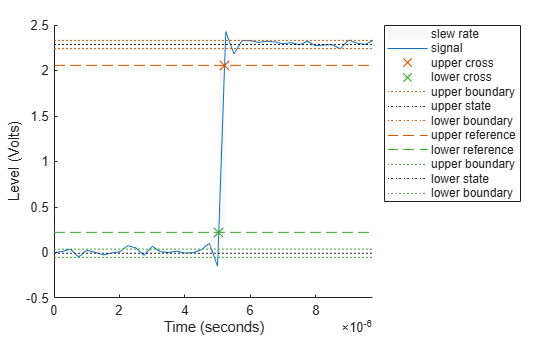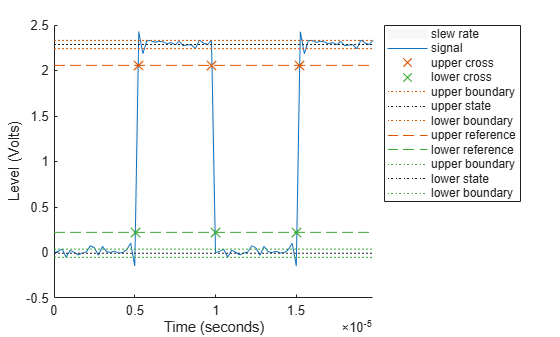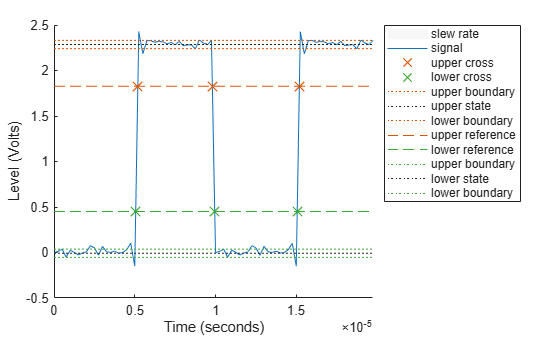# slewrate

Slew rate of bilevel waveform

## Syntax

s = slewrate(x)
s = slewrate(x,Fs)
s = slewrate(x,t)
[s,lt,ut] = slewrate(___)
[s,lt,ut,ll,ul] = slewrate(___)
s = slewrate(___,Name,Value)
slewrate(___)

## Description

example

s = slewrate(x) returns the slew rate for all transitions found in the bilevel waveform x. To determine the transitions, the slewrate function estimates the state levels of the input waveform by a histogram method and identifies all regions that cross the upper-state boundary of the low state and the lower-state boundary of the high state.

example

s = slewrate(x,Fs) specifies the sample rate Fs.

example

s = slewrate(x,t) specifies the sample instants t.

example

[s,lt,ut] = slewrate(___) returns the time instants when the waveform crosses the lower-percent reference level lt and upper-percent reference level ut. If you do not specify lower- and upper-percent reference levels, the levels default to 10% and 90%, respectively. You can specify an input combination from any of the previous syntaxes.

example

[s,lt,ut,ll,ul] = slewrate(___) returns the waveform values that correspond to the lower-reference levels ll and upper-reference levels ul.
s = slewrate(___,Name,Value) returns the slew rate for all transitions with additional options specified by one or more Name,Value arguments.

example

slewrate(___) plots the bilevel waveform and darkens the regions of each transition where the slew rate is computed. The plot marks the lower- and upper-reference level crossings and associated reference levels. The plot also indicates the state levels and associated lower and upper tolerances.

## Examples

collapse all

Use slewrate with no output arguments to plot the slew rate information for a step waveform sampled at 4 MHz.

Load the transitionex.mat file and compute the slew rate. Annotate the slew rate in a plot of the waveform.

load('transitionex.mat','x','t') slewrate(x,t)ans = 1.0310e+07 

Create a bilevel waveform with three transitions, two positive and one negative. The sample rate is 4 MHz. Obtain the slew rates for the three transitions.

load('transitionex.mat','x') fs = 4e6; y = [x;fliplr(x)]; t = (0:length(y)-1)/4e6; S = slewrate(y,t)
S = 3×1 107 × 1.0310 -0.9320 1.0310 

Annotate the result on a plot of the waveform.

slewrate(y,t);Return the lower- and upper-transition times for a three-transition waveform sampled at 4 MHz.

load('transitionex.mat','x') fs = 4e6; y = [x;fliplr(x)]; t = (0:length(y)-1)/fs; [~,LT,UT] = slewrate(y,t)
LT = 3×1 10-4 × 0.0504 0.0998 0.1504 
UT = 3×1 10-4 × 0.0521 0.0978 0.1521 

Repeat using the sample rate instead of the time vector.

[~,LT,UT] = slewrate(y,fs)
LT = 3×1 10-4 × 0.0504 0.0998 0.1504 
UT = 3×1 10-4 × 0.0521 0.0978 0.1521 

Annotate the result on a plot of the waveform.

slewrate(y,fs);Return the waveform values corresponding to the lower- and upper-reference levels for a three-transition waveform sampled at 4 MHz. Compute these values for 10% and 90%, the default levels.

load("transitionex.mat","x") fs = 4e6; y = [x;fliplr(x)]; t = (0:length(y)-1)/fs; [~,~,~,LL,UL] = slewrate(y,t)
LL = 0.2212 
UL = 2.0564 

Repeat the calculation for 20% and 80%. Annotate the result on a plot of the waveform

slewrate(y,t,PercentReferenceLevels=[20 80]);## Input Arguments

collapse all

Bilevel waveform, specified as a real-valued vector. If the input waveform does not have at least one transition, the function returns an empty matrix. The first time instant in x corresponds to t=0.

Sample rate, specified as a positive real scalar in hertz.

Sample instants, specified as a vector. The length of t must equal the length of the input bilevel waveform x. The sample instants correspond to the indices of the input vector.

### Name-Value Arguments

Specify optional pairs of arguments as Name1=Value1,...,NameN=ValueN, where Name is the argument name and Value is the corresponding value. Name-value arguments must appear after other arguments, but the order of the pairs does not matter.

Example: s = slewrate(x,t,Tolerance=5)

Before R2021a, use commas to separate each name and value, and enclose Name in quotes.

Example: s = slewrate(x,t,'Tolerance',5)

Percent reference levels, specified as a 1-by-2 real-valued vector. For more information, see Percent Reference Levels.

Low- and high-state levels, specified as a 1-by-2 real-valued vector. The first element is the low-state level and the second element is the high-state level. If you do not specify StateLevels, the function estimates the state levels from the input waveform using the histogram method.

Tolerance levels (lower and upper state boundaries), specified as a scalar and expressed as a percentage. The low-state and high-state boundaries are expressed as the state level plus or minus a multiple of the difference between the state levels. For more information, see State-Level Tolerances.

## Output Arguments

collapse all

Slew rate, returned as a vector. A positive slew rate indicates that the upper-percent reference level occurs later than the lower-percent reference level. A negative slew rate indicates that the upper-percent reference level occurs before the lower-percent reference level. The slew rate is the slope of the line connecting the 10% and 90% reference levels.

Time instants when the input signal crosses the lower percent reference level, returned as a vector.

Time instants when the input signal crosses the upper-percent reference level, returned as a vector.

Waveform values at the lower-percent reference level, returned as a vector.

Waveform values at the upper-percent reference level, returned as a vector.

collapse all

### Percent Reference Levels

If S1 is the low state, S2 is the high state, and U is the upper-percent reference level, then the waveform value corresponding to the upper-percent reference level is

${S}_{1}+\frac{U}{100}\left({S}_{2}-{S}_{1}\right).$

If L is the lower-percent reference level, the waveform value corresponding to the lower percent reference level is

${S}_{1}+\frac{L}{100}\left({S}_{2}-{S}_{1}\right).$

### Slew Rate

The slew rate is the slope of a line connecting the upper- and lower-percent reference levels. Let tL denote the time instant when the waveform crosses the lower reference level and tU denote the time instant when the waveform crosses the upper percent reference level. Using the definitions for the upper and lower percent reference levels given in Percent Reference Levels, the slew rate is

$\frac{{S}_{1}+\frac{U}{100}\left({S}_{2}-{S}_{1}\right)-\left\{{S}_{1}+\frac{L}{100}\left({S}_{2}-{S}_{1}\right)\right\}}{{t}_{U}-{t}_{L}}=\frac{U-L}{100}\frac{{S}_{2}-{S}_{1}}{{t}_{U}-{t}_{L}}.$

When tL occurs earlier than tU, the slew rate is positive. When tU occurs earlier than tL, the slew rate is negative.

### State-Level Tolerances

You can specify lower- and upper-state boundaries for each state level. Define the boundaries as the state level plus or minus a scalar multiple of the difference between the high state and the low state. To provide a useful tolerance region, specify the scalar as a small number such as 2/100 or 3/100. In general, theregion for the low state is defined aswhereis the low-state level andis the high-state level. Replace the first term in the equation withto obtain thetolerance region for the high state.

This figure shows lower and upper 5% state boundaries (tolerance regions) for a positive-polarity bilevel waveform. The thick dashed lines indicate the estimated state levels.IEEE® Standard on Transitions, Pulses, and Related Waveforms, IEEE Standard 181, 2003.